# Physics Engine

• It is the final project for the course of Fundamentals of Computer Aided Design, THU 2018 Fall, programming with OpenGL.
• It’s a course assignment of a Computer Aided Design couse in CMU.
• The source code can be found on my github page. The other programming assignments of this course are also maintained in the repository. The detailed instruction is in addtionalMaterial.pdf and README. The source edited files are src/physics/collision*, physics*, spherebody*, spring*.
• I was also selected to give a presentation about my project.

### Demo

There are 6 scenes to be implemented in this project.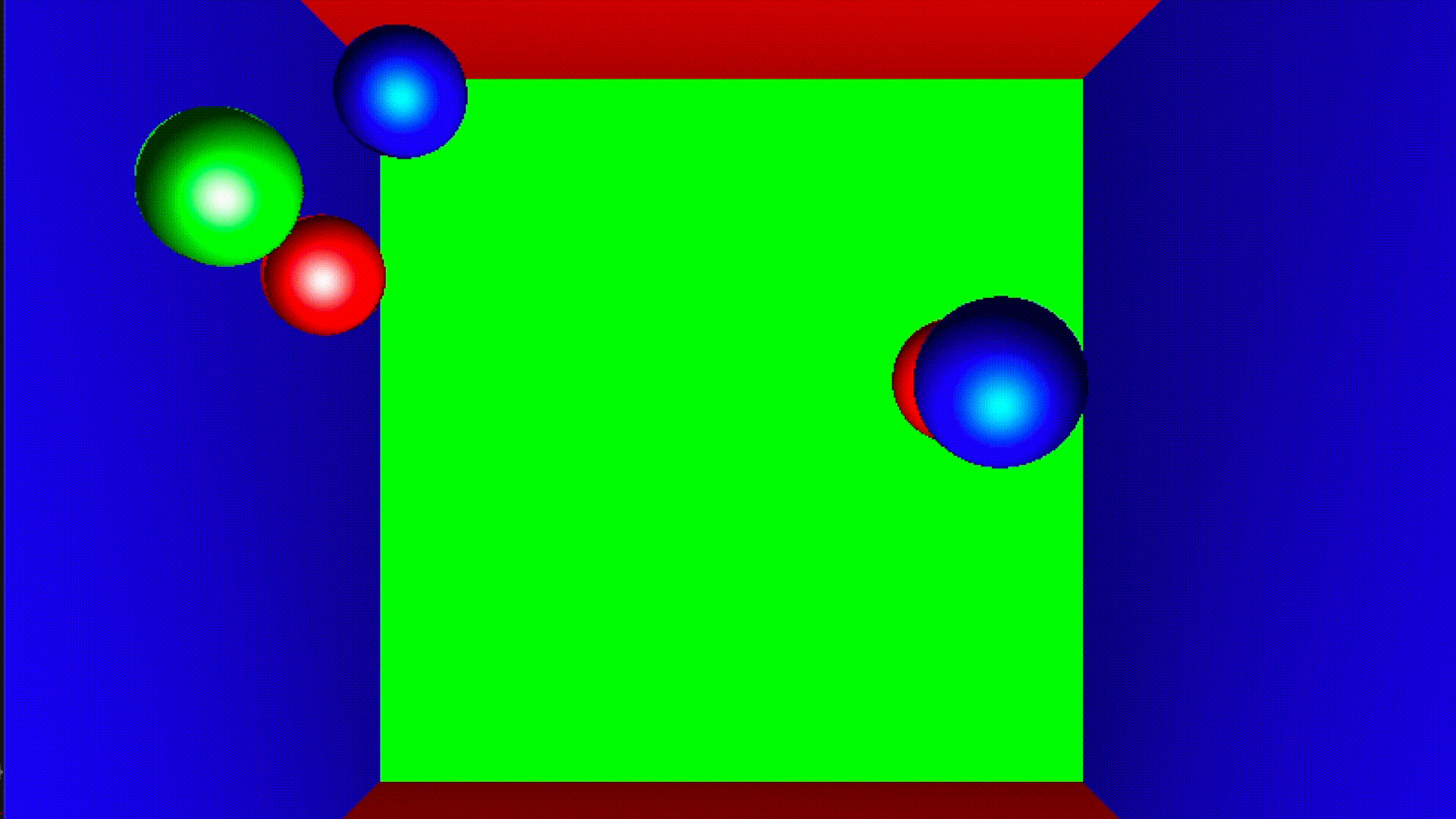Collision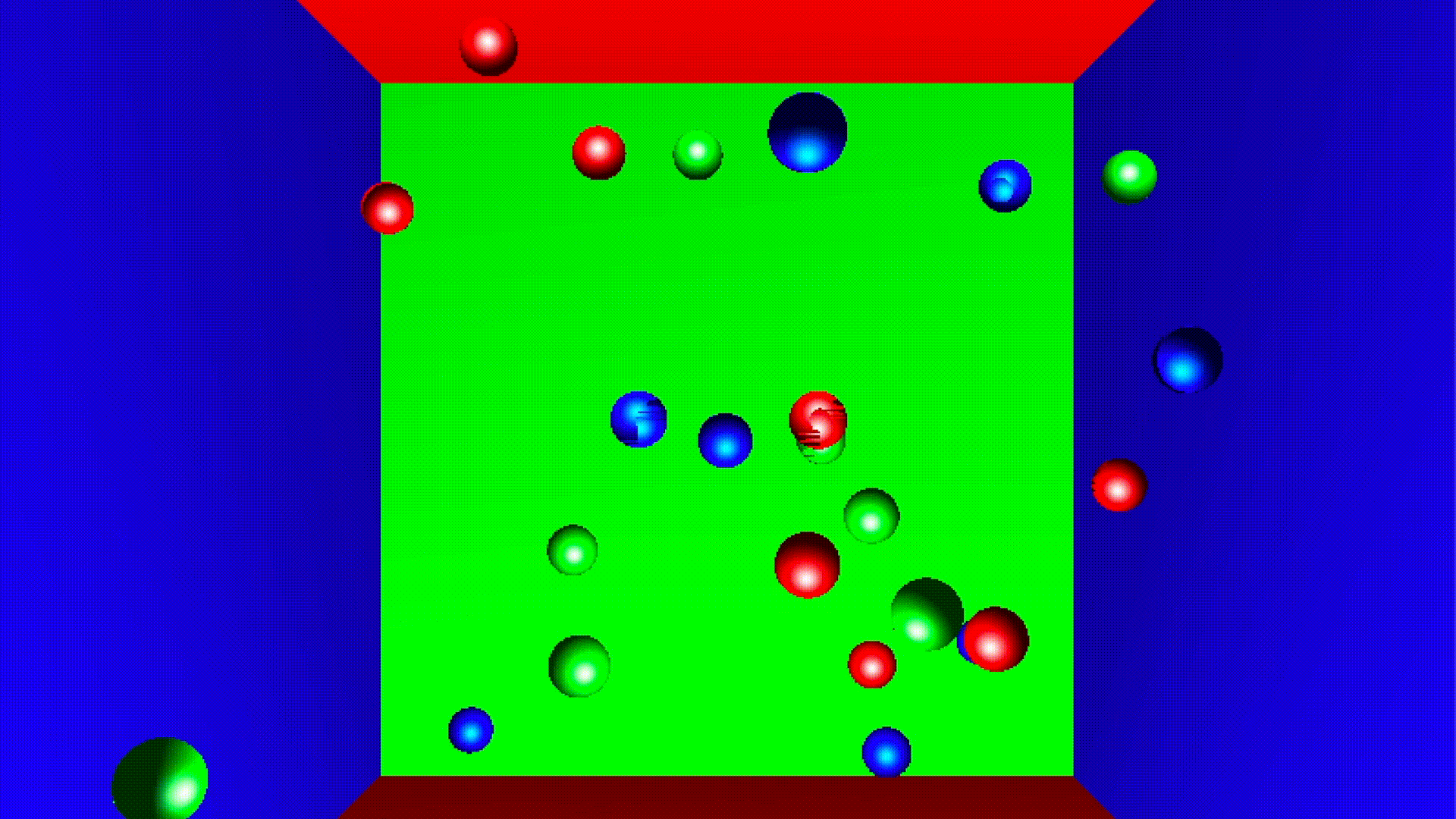Collision Stress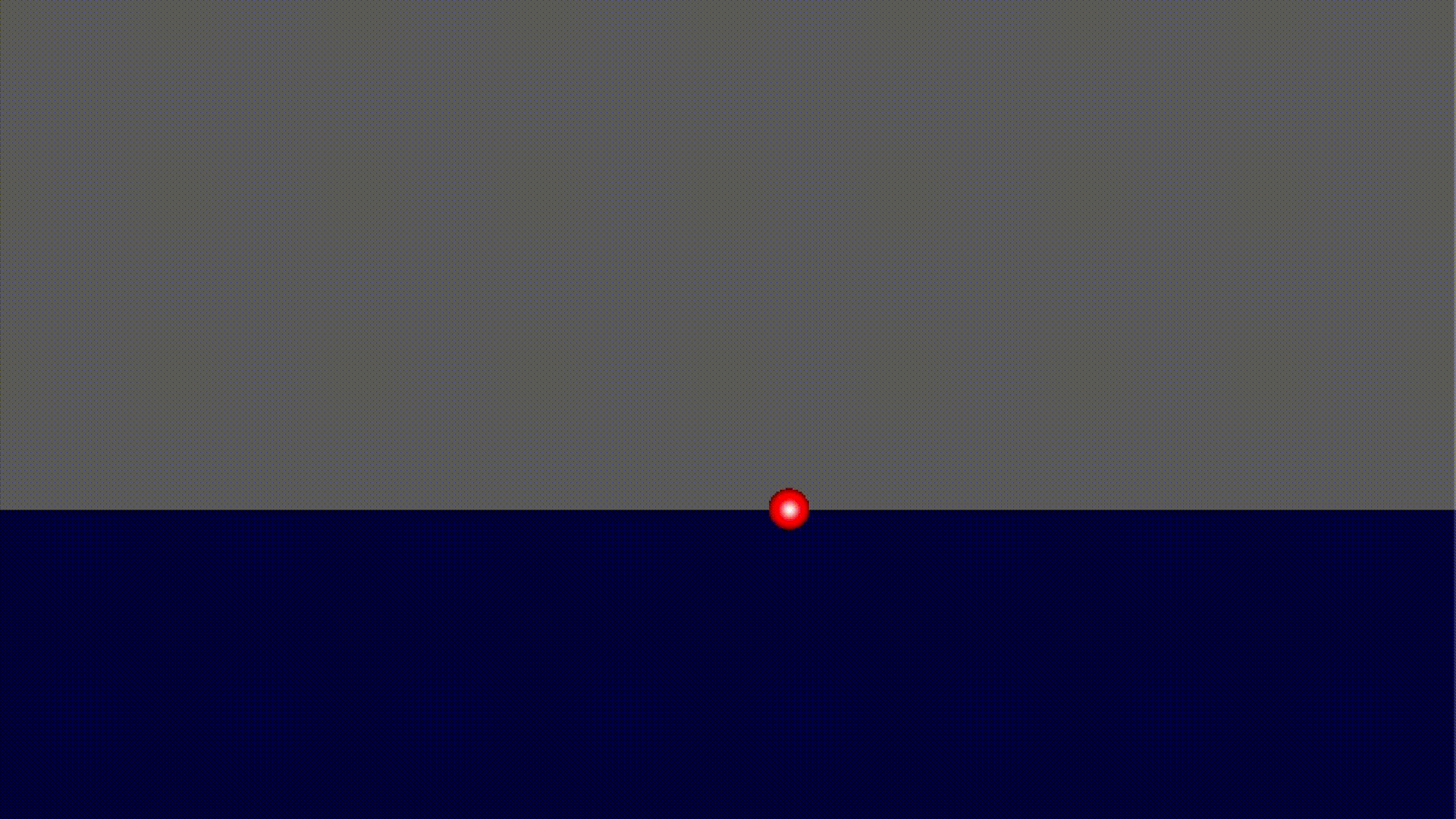Damping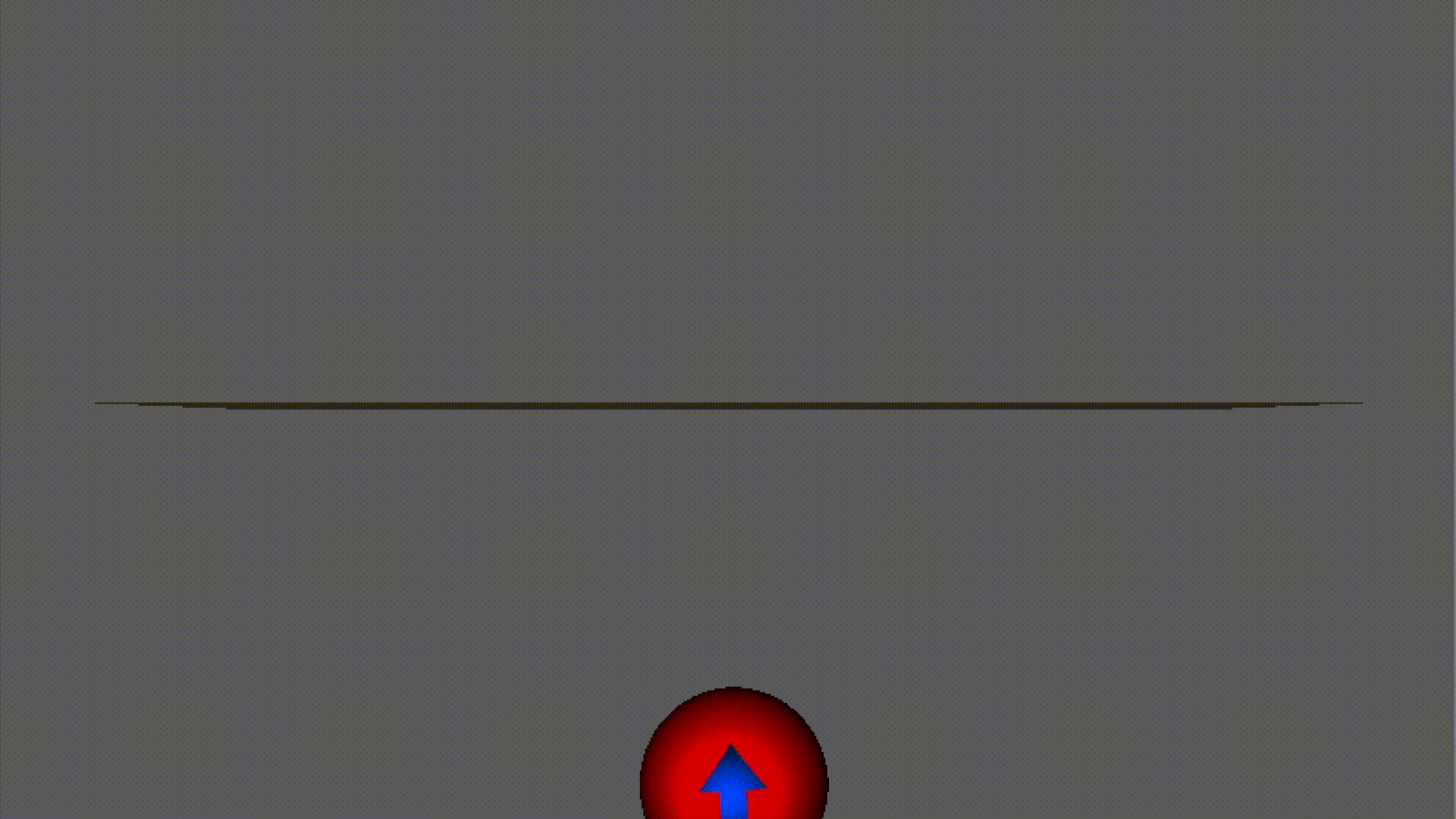Spring Rotation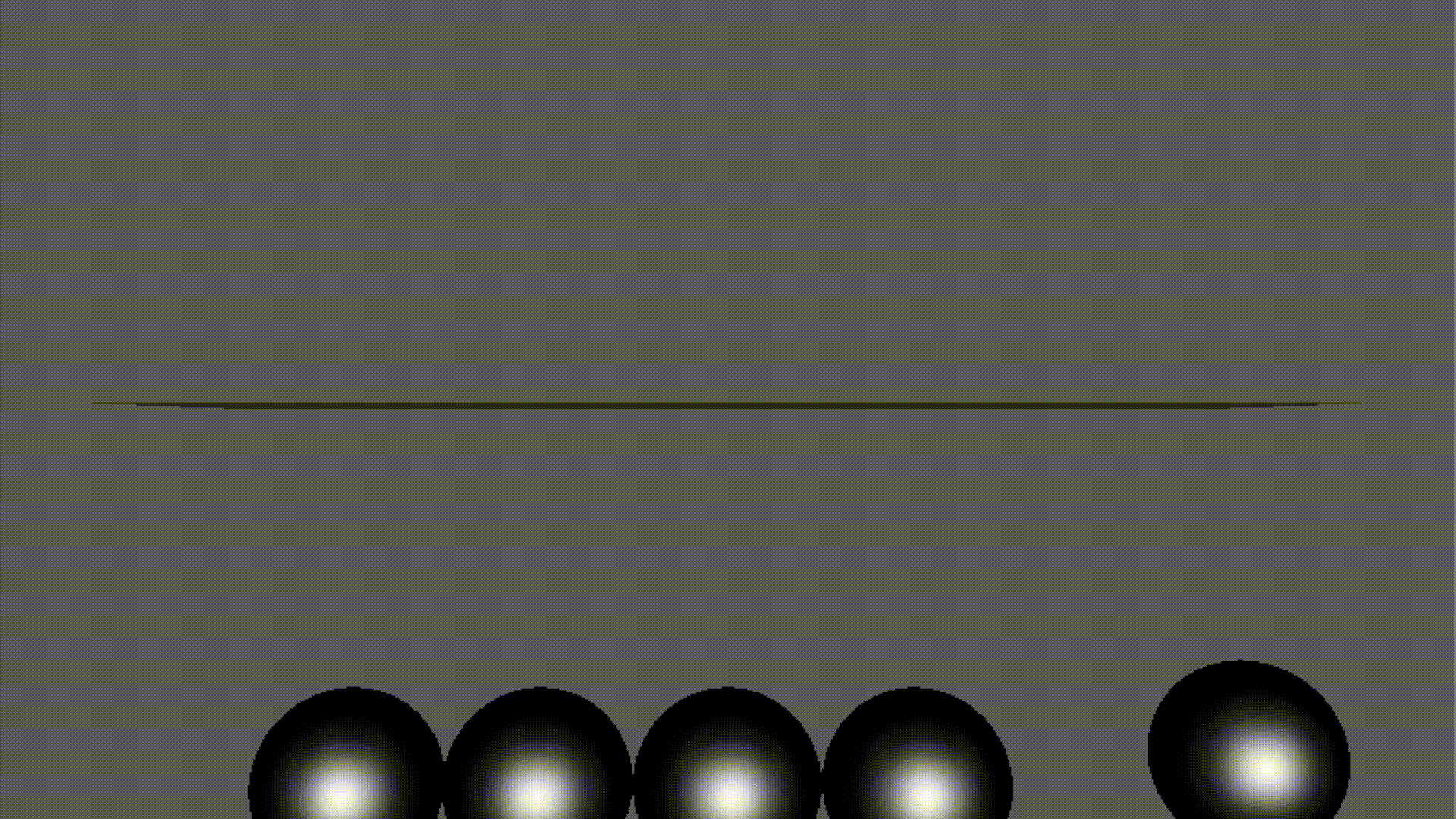Newtons CradleRotation

### Implement Details

• Collision — Detection

• Sphere & Sphere: A collision would happen when $\overrightarrow{O_1 O_2} < r_1+r_2$ and $(\vec{v}_1 - \vec{v}_2)\cdot\overrightarrow{O_1O_2} >0$.
• Sphere & Plane: d<r and sphere moves towards the plane.
• Sphere & Triangle: When the projection of the sphere center on the triangle’s plane falls in the triangle, i.e., $(\overrightarrow{PA_0} \times \overrightarrow{PA_1}), (\overrightarrow{PA_1}\times \overrightarrow{PA_2}), (\overrightarrow{PA_2}\times \overrightarrow{PA_0})$ have the same orientation, the same as the plane case. Otherwise, consider the projection of P on the three edges (if falls outside, the select the nearest vertex). Calculate the distance of the three selected points to the sphere center respectively; one of them is the nearest distance of the two bodies, which is also the collision point if collision happens.
• Spring — Force Apply

• The step() function applies forces and torques to the attached bodies.
• The force is applied every frame, so we should clear the force applied in the previous frame.
• SphereBody — Integration

• The force varies with velocity and position. So we need to use 2-degree RK4 in Physics and call the Spring::step to update force (acceleration). The motion_damping used in SphereBody‘s step functions also need to be updated by Spring, which is a little troublesome. I just see force as a constant in every frame.
• I was confused by the requirements in the given files, so I did not really realize the RK4 method. The time was limited to complete this task, so I used my friends’ method —- Although this method actually equals to $\begin{cases} v = v_0 + dt \cdot a \\ x = dt \cdot v_0 + \frac{1}{2} a\cdot dt^2 \end{cases}$, if use the latter directly, the error could not be neglected and would finally causes huge deviation. That is really a strange phenomenon and I have lost the code with the latter method. The only explanation to this I could find is the calculating precision of this data type.
• Physics — Move Forward

• The position and angular_position of a SphereBody::Sphere has already been updated in SphereBody, so in Physics the only thing to do is to call them. The gravity is applied in the initialization.
• Fisrt, check collisions by traverse the sphere list and check them with the other bodies (including sphere).
• Traverse the spring list, apply forces.
• Traverse all the sphere and update them.

### Environment & Dependencies

• MacOS Mojave
• OpenGL and other framework
• SDL1.2 (not 2.0)
• libpng-1.2.57 (this version only)
• GLEW, GLUT
(The makefile may need to be changed under other operation system)

### Other Assignments

• The course also has 7 other assignments, six of which are programming work (the other one is a design work, and I even forget to hand in my design).
• OpenGL can achieve a pretty beautiful effect so I put all of them here.
• My work in Solar System was also selected as one of the two excellent homework by the TA.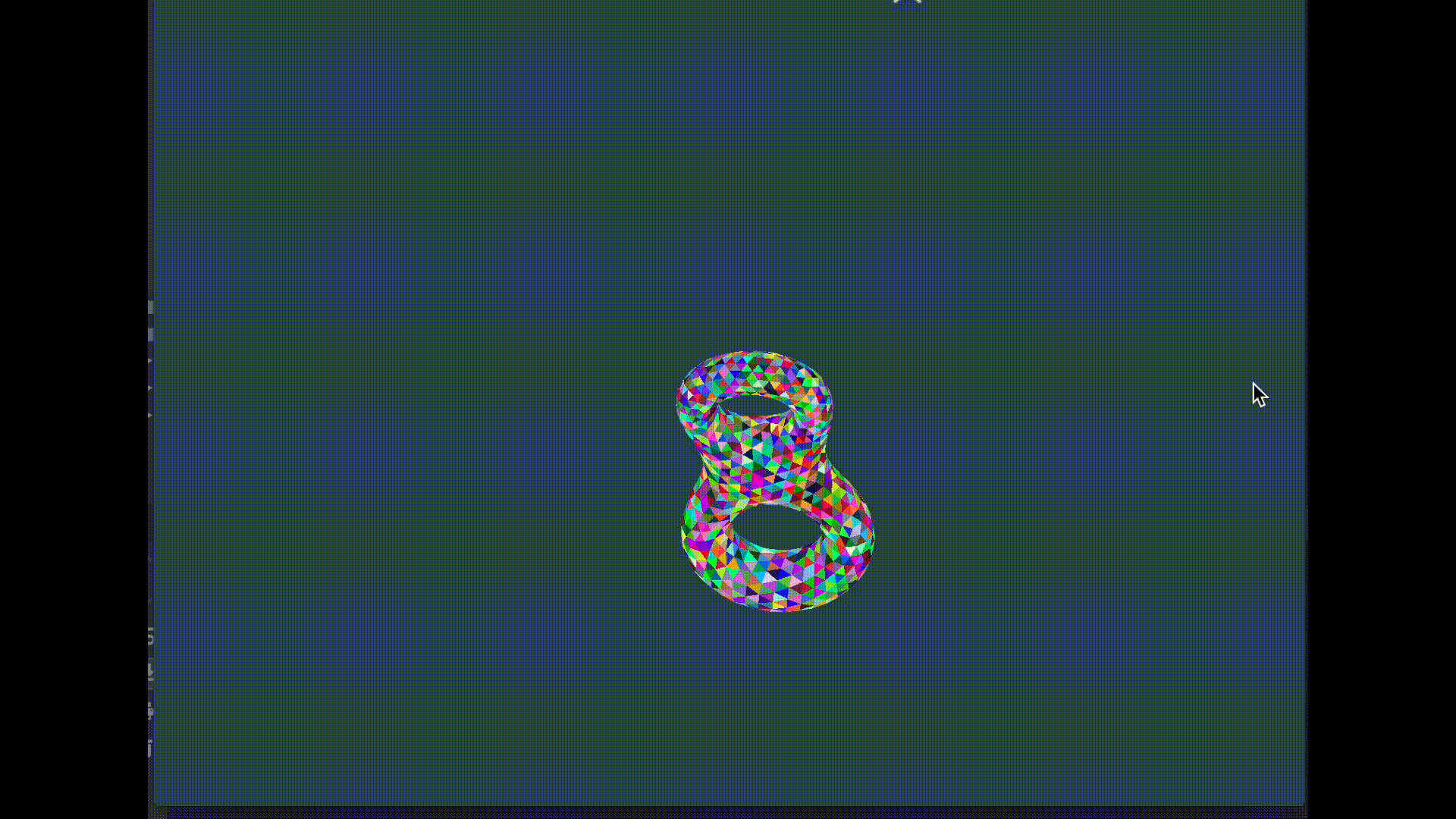Display a 3D Model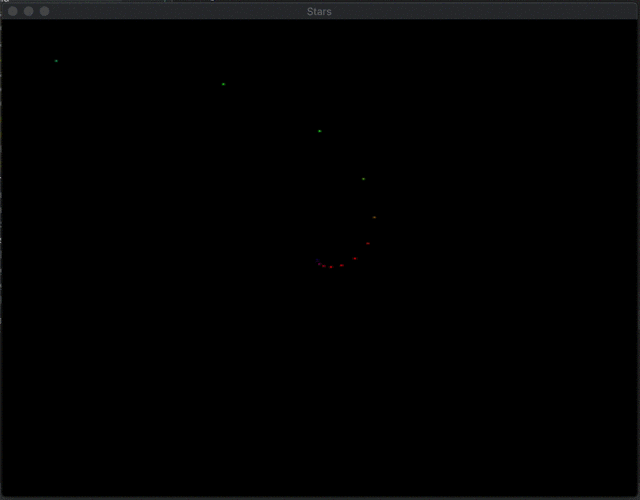Rotating Stars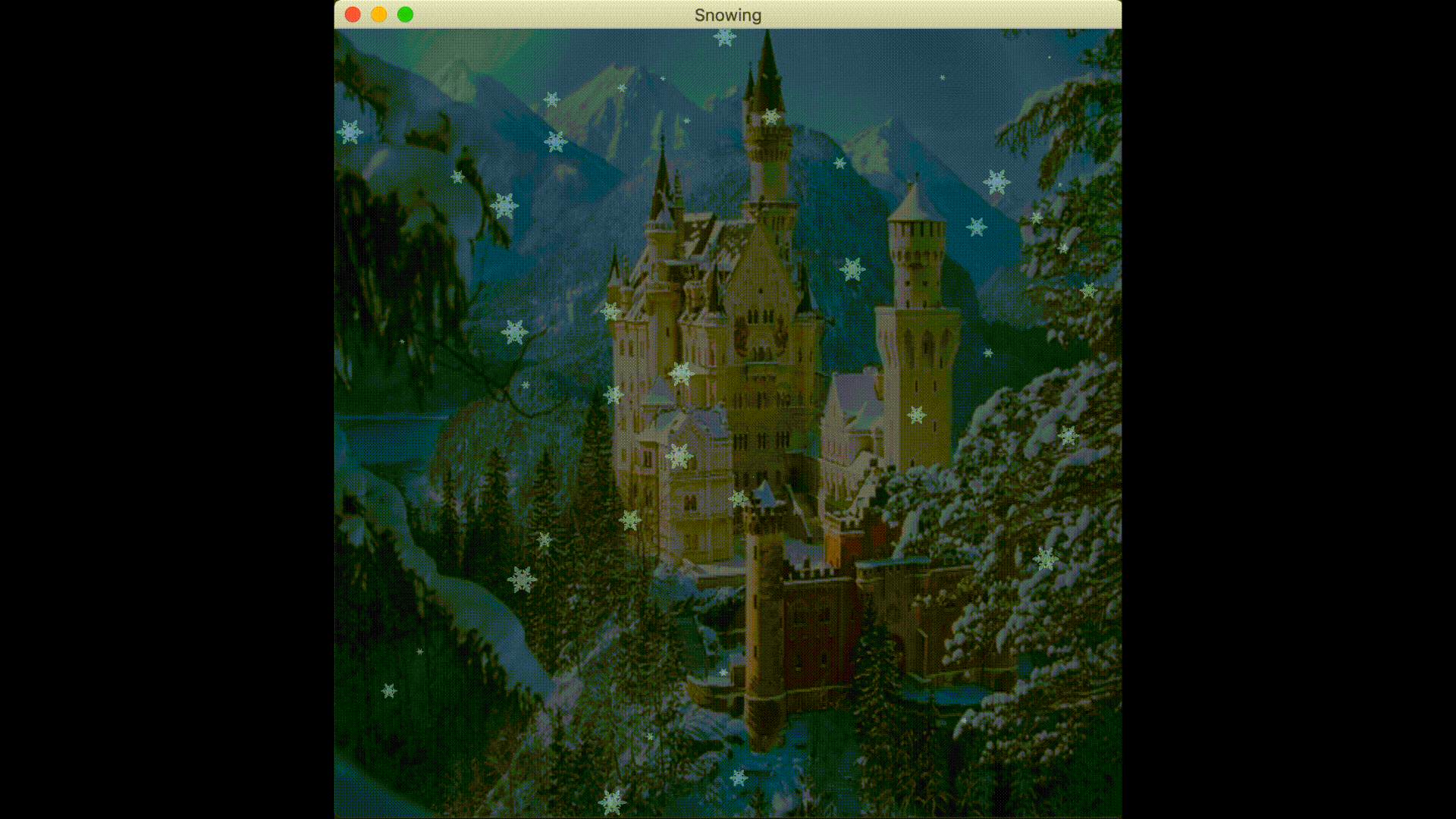Snow Falling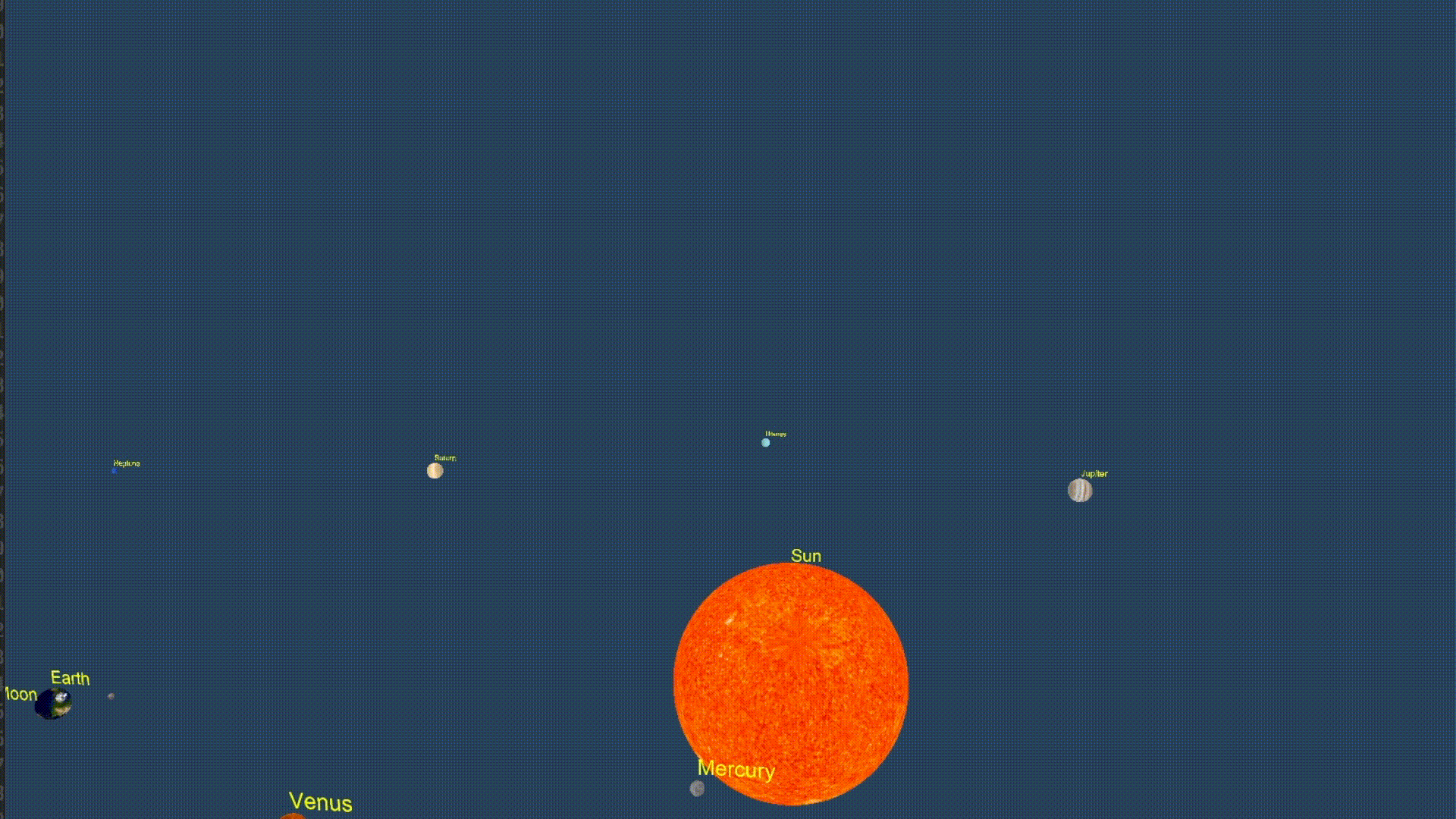Solar System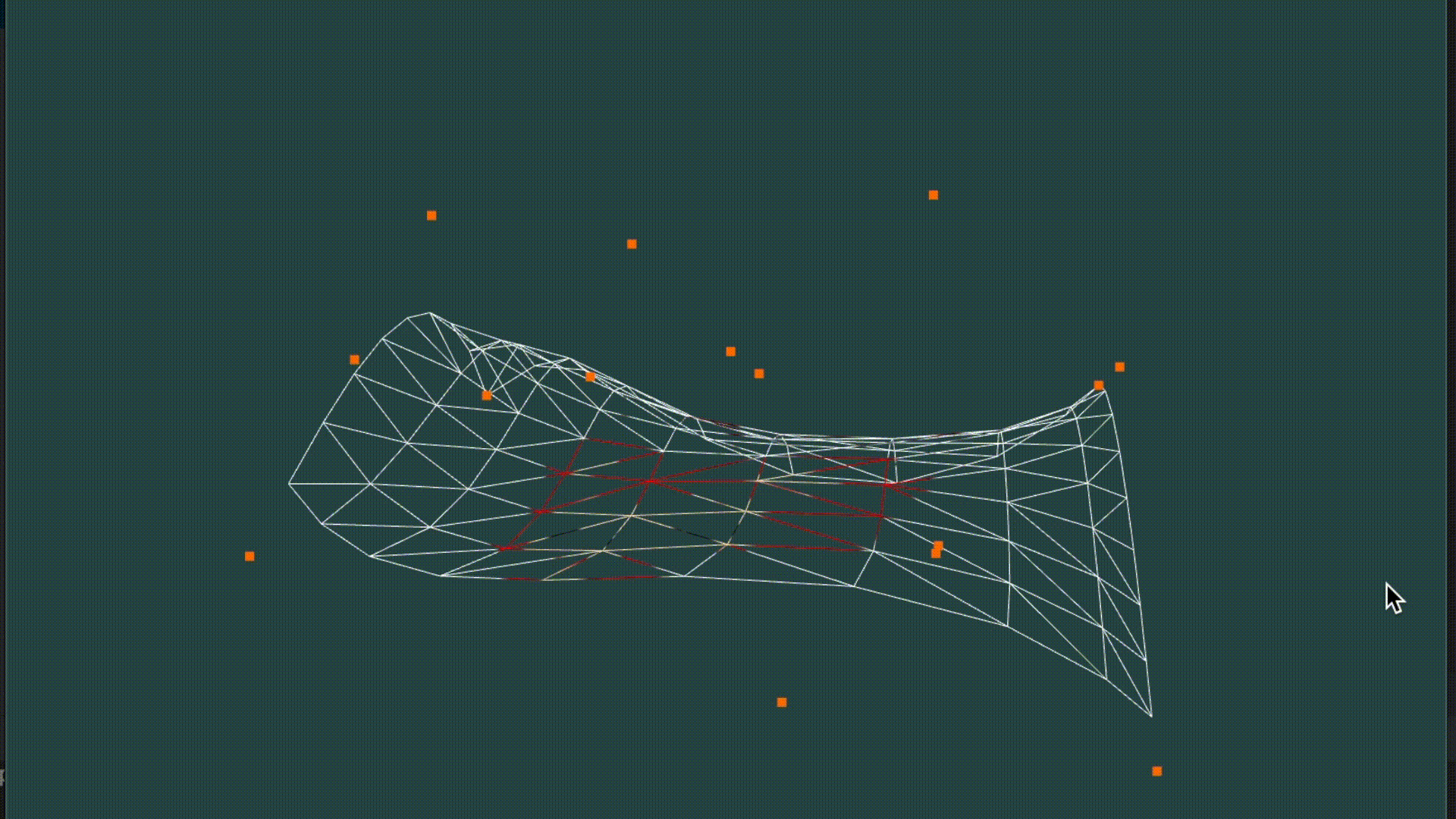Bezier Surface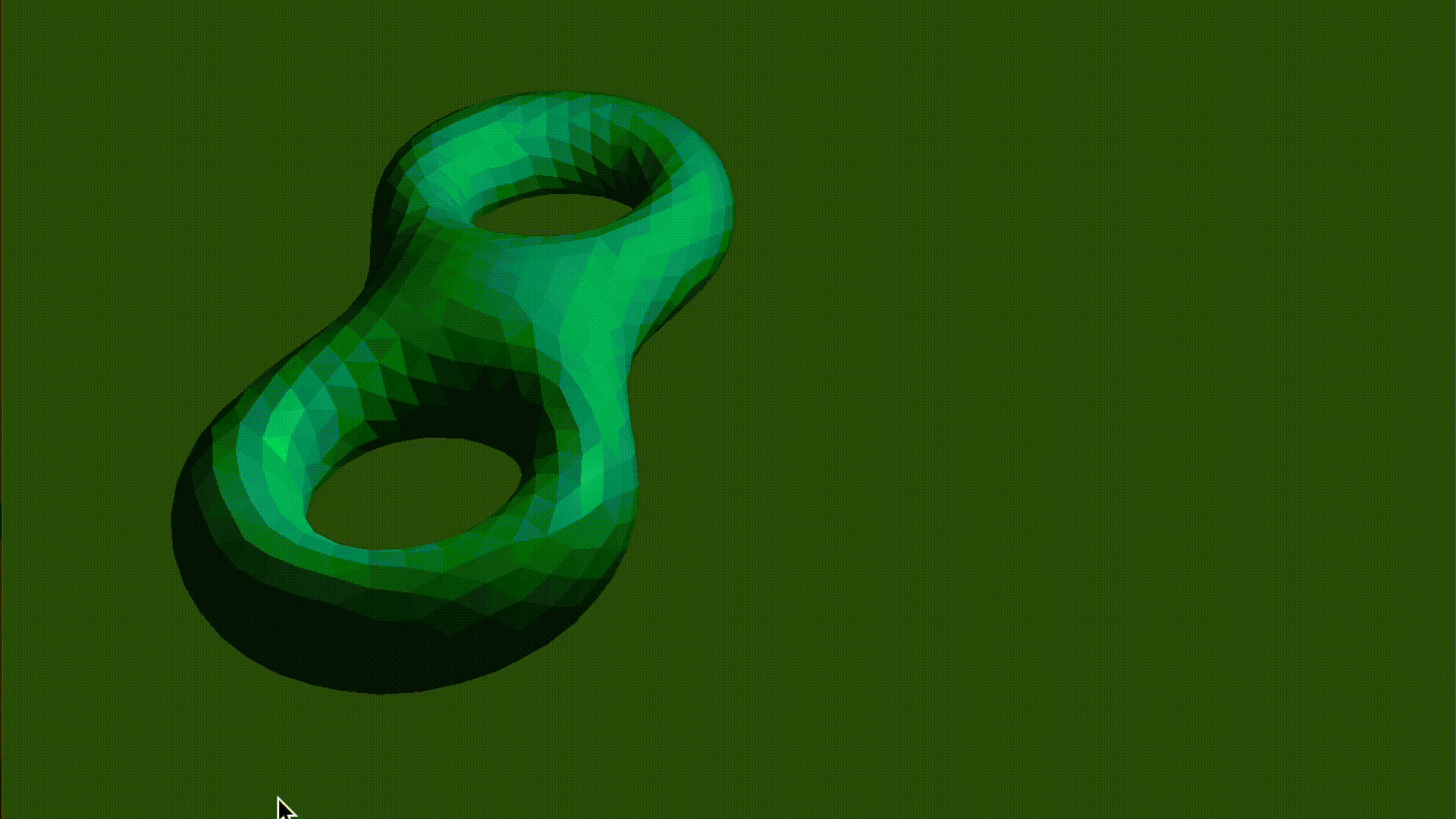Lighting the 3D Model

### Acknowledgment

• The basic frame of those works was given by TA.
• Thanks for the help of my senior Rui Wang and my classmate Ran Zhou.
Share